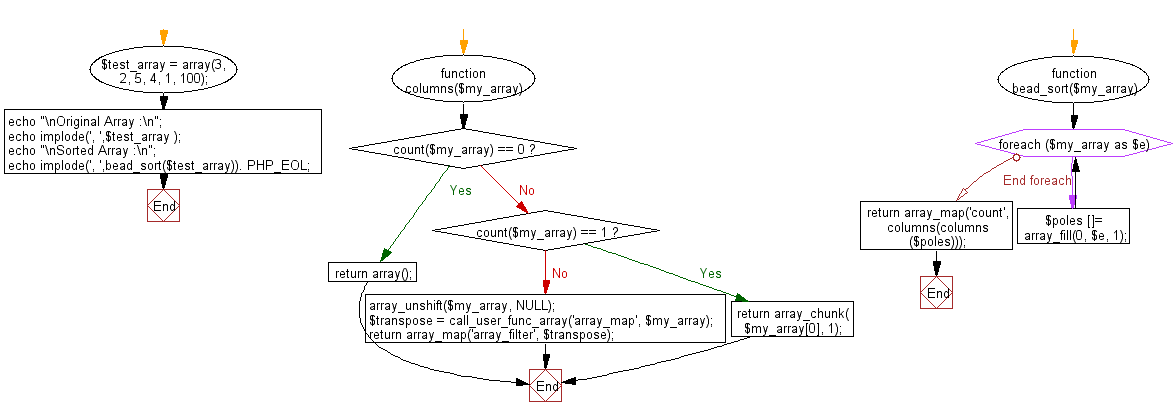﻿ PHP: Sort a list of elements using Bead sort - w3resource

# PHP Searching and Sorting Algorithm: Bead sort

## PHP Searching and Sorting Algorithm: Exercise-13 with Solution

Write a PHP program to sort a list of elements using Bead sort.

Sample Solution :

PHP Code :

``````<?php
function columns(\$my_array) {
if (count(\$my_array) == 0)
return array();
else if (count(\$my_array) == 1)
return array_chunk(\$my_array, 1);
array_unshift(\$my_array, NULL);
// array_map(NULL, \$my_array, \$my_array, ...)
\$transpose = call_user_func_array('array_map', \$my_array);
return array_map('array_filter', \$transpose);
}
foreach (\$my_array as \$e)
\$poles []= array_fill(0, \$e, 1);
return array_map('count', columns(columns(\$poles)));
}
\$test_array = array(3, 2, 5, 4, 1, 100);
echo "\nOriginal Array :\n";
echo implode(', ',\$test_array );
echo "\nSorted Array :\n";
```
?>
```

Sample Output:

```Original Array
:3, 2, 5, 4, 1, 100
Sorted Array :
100, 5, 4, 3, 2, 1
```

Flowchart :PHP Code Editor:

Have another way to solve this solution? Contribute your code (and comments) through Disqus.

What is the difficulty level of this exercise?

Test your Programming skills with w3resource's quiz.

﻿